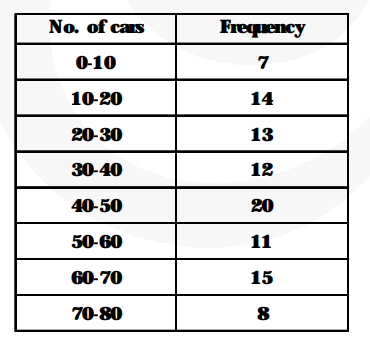# A student noted the number of cars passing through a spot on a road for 100 periods each of 3 minutes and summarised it in the table given below.`
Question:

A student noted the number of cars passing through a spot on a road for 100 periods each of 3 minutes and summarised it in the table given below. Find the mode of the data.Solution:

Modal class = 40 – 50

Mode $=40+\left\{\frac{\mathbf{2 0}-\mathbf{1 2}}{\mathbf{2} \times \mathbf{2 0}-\mathbf{1 2}-\mathbf{1 1}}\right\} \times 10=40+\left\{\frac{\mathbf{8}}{\mathbf{4 0}-\mathbf{2 3}}\right\} \times 10$

$=40+4.706=44.706$